# Multivariate polynomial ring over a field

## Definition

The multivariate polynomial ring over a field is defined as a polynomial ring in (finitely) many variables over a field. If we denote the underlying field by$k$, and the variables by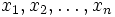$x_1,x_2,\ldots,x_n$, then the polynomial ring is denoted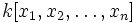$k[x_1,x_2,\ldots,x_n]$.

## Properties

The multivariate polynomial ring over a field is a unique factorization domain as well as a Noetherian domain. When there is more than one variable, it is not a Euclidean domain or a principal ideal domain.

## Extra structure

Further information: multivariate polynomial ring#extra structure

The multivariate polynomial ring$k[x_1,x_2,\ldots,x_n]$ can be viewed as a$k$-algebra. It also has the following additional structures:

• Graded ring structure: In particular, it is a graded$k$-algebra where the$d^{th}$ component is the vector space generated by monomials of degree$d$
• Filtered ring structure: In particular, it is a filtered$k$-algebra where the$d^{th}$ filtration is the subspace of polynomials of degree at most$d$

## Ideals and quotients

Quotients of the polynomial ring in$n$ variables, are precisely the same as algebras generated by$n$ elements as$k$-algebras. The structure of these is in general fairly complicated.

## Automorphisms and endomorphisms

### As an algebra over the base field

The multivariate polynomial ring$k[x_1,x_2,\ldots,x_n]$ can be viewed as a$k$-algebra. The endomorphisms with respect to this structure are the endomorphisms that fix the elements of$k$.

• The endomorphisms are in bijective correspondence with sequences of polynomials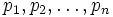$p_1,p_2,\ldots,p_n$. Here$p_i$ is the polynomial to which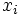$x_i$ goes under the endomorphisms. Composition of endomorphisms is by composing the polynomials.
• The automorphisms are the multiplicative subgroup of this monoid of endomorphisms described above. In general, there is no easy or direct description by which one can test whether a given endomorphism is an automorphism.

### As a filtered ring

The multivariate polynomial ring$k[x_1,x_2,\ldots,x_n]$ has a filtration, where the$d^{th}$ level of the filtration comprises all polynomials of degree at most$d$. The endomorphisms for this filtration are thus endomorphisms that are degree-preserving.

• In particular, the endomorphisms preserve the first level of the filtration, i.e. they send linear polynomials to linear polynomials. This shows that the monoid of endomorphisms is naturally identified with the affine maps from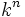$k^n$ to$k^n$ i.e. maps that preserve collinearity and ratios of distances along a line.
• The automorphisms are identified with the general affine group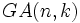$GA(n,k)$, the group of collinearity-cum-ratio preserving bijective maps from$k^n$ to$k^n$.

The multivariate polynomial ring$k[x_1,x_2,\ldots,x_n]$ has a gradation, where the$d^{th}$ graded component is generated over$k$ by the monomials of total degree$d$. The endomorphisms that preserve this gradation must send homogeneous linear polynomials to homogeneous linear polynomials.
• In particular, the endomorphism monoid is the multiplicative monoid of the matrix ring$M(n,k)$
• The automorphism group is the general linear group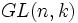$GL(n,k)$